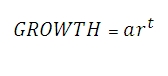# SQL Server GROWTH function

GROWTH_q

Updated: 9 August 2010

Use GROWTH_q to calculate predicted exponential growth by using existing data. GROWTH returns the y-value for a new x-value that you specify using existing x-values and y-values.Where
a = 10m
r = 10b
b = SLOPE(log(known_y) / log(10), known_x)
m = INTERCEPT(log(known_y) / log(10), known_x)
t = new x
Syntax
SELECT [wctStatistics].[wct].[GROWTH_q] (
<@Known_y_Known_x_RangeQuery, nvarchar(4000),>
,<@New_x, float,>)
Arguments

@Known_y_Known_x_RangeQuery
the select statement, as text, used to determine the known y- and x-values to be used in the GROWTH_q calculation.
@New_x
the new x-value for which you want GROWTH to calculate the y-value.
Return Types
float
Remarks
·         If the number of y-data points is not equal to the number of x-data points, GROWTH will return an error.
·         If any of the values in the known-y dataset is less than or equal to zero, GROWTH returns an error
·         No GROUP BY is required for this function even though it produces aggregated results.
Examples

Using the de-normalized table #f2, project the sales for MSFT in 2009

select 'MSFT' as [Ticker]
,wct.GROWTH_q('Select SALES, FY from #f2 where SYMBOL = ' + CHAR(39) + 'MSFT' + CHAR(39), 2009) as [2009 Sales]

This produces the following result

Ticker 2009 Sales
------ ----------------------
MSFT   65495.2084347367

(1 row(s) affected)

Using the de-normalized table #f2, calculate the EPS for MSFT for the next 6 years.

with mycte as
(
Select 2009 as FY
UNION ALL
Select FY + 1
from mycte
where FY < 2014
)
select 'MSFT' as [Ticker]
,mycte.FY as [FY]
,wct.GROWTH_q(
'Select EPS
,FY
from #f2
where SYMBOL = ' + CHAR(39) + 'MSFT' + CHAR(39)
,mycte.FY) as [EPS]
from mycte

This produces the following result

Ticker FY          EPS
------ ----------- ----------------------
MSFT   2009        1.63289654265364
MSFT   2010        1.81780539503368
MSFT   2011        2.02365328598437
MSFT   2012        2.25281134771825
MSFT   2013        2.50791921894833
MSFT   2014        2.79191545050624

(6 row(s) affected)

### SupportCopyright 2008-2021 Westclintech LLC         Privacy Policy        Terms of Service